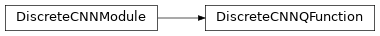# garage.torch.q_functions.discrete_cnn_q_function¶

Discrete CNN Q Function.

class DiscreteCNNQFunction(env_spec, kernel_sizes, hidden_channels, strides, hidden_sizes=(32, 32), cnn_hidden_nonlinearity=torch.nn.ReLU, mlp_hidden_nonlinearity=torch.nn.ReLU, hidden_w_init=nn.init.xavier_uniform_, hidden_b_init=nn.init.zeros_, paddings=0, padding_mode='zeros', max_pool=False, pool_shape=None, pool_stride=1, output_nonlinearity=None, output_w_init=nn.init.xavier_uniform_, output_b_init=nn.init.zeros_, layer_normalization=False, is_image=True)Discrete CNN Q Function.

A Q network that estimates Q values of all possible discrete actions. It is constructed using a CNN followed by one or more fully-connected layers.

Parameters
• env_spec (EnvSpec) – Environment specification.

• kernel_sizes (tuple[int]) – Dimension of the conv filters. For example, (3, 5) means there are two convolutional layers. The filter for first layer is of dimension (3 x 3) and the second one is of dimension (5 x 5).

• strides (tuple[int]) – The stride of the sliding window. For example, (1, 2) means there are two convolutional layers. The stride of the filter for first layer is 1 and that of the second layer is 2.

• hidden_channels (tuple[int]) – Number of output channels for CNN. For example, (3, 32) means there are two convolutional layers. The filter for the first conv layer outputs 3 channels and the second one outputs 32 channels.

• hidden_sizes (list[int]) – Output dimension of dense layer(s) for the MLP for mean. For example, (32, 32) means the MLP consists of two hidden layers, each with 32 hidden units.

• mlp_hidden_nonlinearity (callable) – Activation function for intermediate dense layer(s) in the MLP. It should return a torch.Tensor. Set it to None to maintain a linear activation.

• cnn_hidden_nonlinearity (callable) – Activation function for intermediate CNN layer(s). It should return a torch.Tensor. Set it to None to maintain a linear activation.

• hidden_w_init (callable) – Initializer function for the weight of intermediate dense layer(s). The function should return a torch.Tensor.

• hidden_b_init (callable) – Initializer function for the bias of intermediate dense layer(s). The function should return a torch.Tensor.

• padding_mode (str) – The type of padding algorithm to use, either ‘SAME’ or ‘VALID’.

• max_pool (bool) – Bool for using max-pooling or not.

• pool_shape (tuple[int]) – Dimension of the pooling layer(s). For example, (2, 2) means that all the pooling layers are of the same shape (2, 2).

• pool_stride (tuple[int]) – The strides of the pooling layer(s). For example, (2, 2) means that all the pooling layers have strides (2, 2).

• output_nonlinearity (callable) – Activation function for output dense layer. It should return a torch.Tensor. Set it to None to maintain a linear activation.

• output_w_init (callable) – Initializer function for the weight of output dense layer(s). The function should return a torch.Tensor.

• output_b_init (callable) – Initializer function for the bias of output dense layer(s). The function should return a torch.Tensor.

• layer_normalization (bool) – Bool for using layer normalization or not.

• is_image (bool) – If true, the inputs are normalized by dividing by 255.

forward(self, observations)

Return Q-value(s).

Parameters

observations (np.ndarray) – observations of shape :math: (N, O*).

Returns

Output value

Return type

torch.Tensor